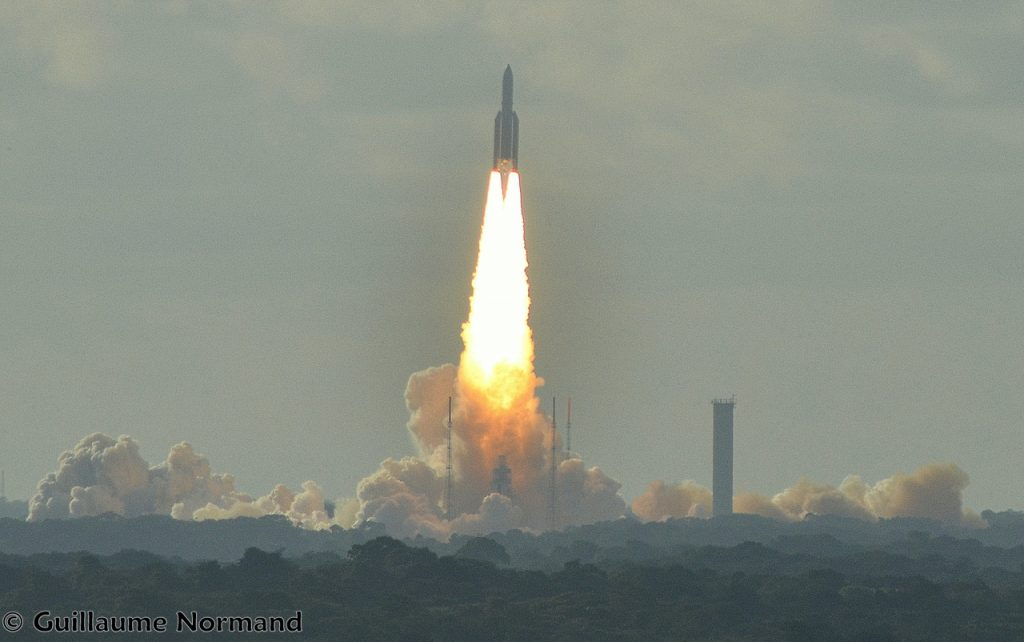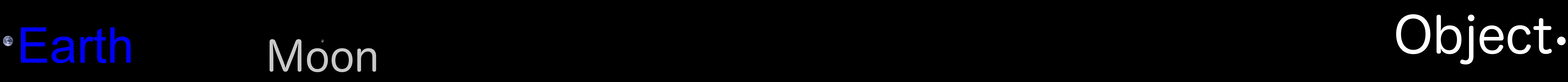# Get into Orbit Without Lateral Acceleration

· Timo Denk

When I was about 6 years old, my dad explained to me that there was no possibility to shoot something right into orbit from the Earth’s surface. He said it was always necessary to accelerate sideways to reach orbit. At that time I did not really understand what he meant. However, later in physics class I did and I figured what he had told me was true. Now, 13 years later, I came up with a new thought: The Earth’s rotation adds lateral velocity to objects launched from its surface. This is also the reason why satellites, the ISS and pretty much every artificial object flying around Earth, orbits Earth counterclockwise and also, why most space flight organizations / companies launch their spacecrafts from locations as close to the equator as possible.The European Space Agency (ESA) has its own space center in Guiana – close to the equator but far away from all the member states. Copyright: Guillaume Normand

As I learned in school there is a fixed velocity for every orbit and vice-versa. In this post I don’t consider non-circular orbits and I don’t take other celestial bodies into account (e.g. including the moon for gravity assists changes the entire thought experiment significantly and increases complexity.) With the fixed correlation of orbit radius $r$ and orbital velocity $v$ the question is: What would be the orbit radius of an object launched from Earth’s equator without any lateral acceleration, but with the initial lateral velocity? Here is the math:

First of all we need the Earth’s radius $r_o$ to calculate the velocity $v$ of objects on the surface:

$$r_0=6.371\times 10^6m\\ U=2\cdot\pi\cdot r_0=40.075\times 10^6m\\ v=\frac{s}{t}=\frac{40.075\times 10^6m}{86164.098s}=465.101\frac{m}{s}$$

Objects on Earth’s equator have a velocity of $v=465.101\frac{m}{s}$ on sea-level. That equals approx. $1674\frac{km}{h}$ ($1040mph$).

Now we can calculate the corresponding circular orbit with radius $r$ (not to be confused with Earth’s radius $r_0$), with $m_0$ being the Earth’s mass:

$$a(r)=\frac{G\cdot m_0}{r^2}\\ v(r)=\sqrt{r\cdot a(r)}=\sqrt{\frac{G\cdot m_0}{r}}\\ r=\frac{G\cdot m_0}{v^2}=1.843\times 10^9m$$

# 1,843,000 km

That’s pretty far away! 1,843,000 km. For comparison: Our moon orbits at 384,400 km.Earth and moon seem to be pretty close together when looking from that far.

So would it be possible to shoot something into a circular orbit at 1.8 million km around Earth by accelerating only upwards? Seems so – but the answer is: No.

Why? Because $r=1.843\times 10^9m$ is outside of the Earth’s sphere of influence (SOI) which has a radius of $r=0.924\times 10^9m$. At that radius the Earth wouldn’t be the primary gravitational influence on the orbiting object. The Sun would be!

My dad was right: It’s not possible to shoot something right into Earth’s orbit without any lateral acceleration. However, this is not necessarily true for all celestial objects out there.

Featured Image